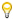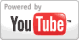Graphing Quadratic Functions - Algebra Lesson
Grade: 7 - 12 | Introduction to the parent graph for quadratic functions: y = x^2. Students then learn how to graph quadratic functions, such as y = x^2 + 2, and how to identify the vertex, minimum, x- and y-intercepts, axis of symmetry, one pair of symmetric points, and the domain and range of the graph.Add to My DashboardAlgebra
For Other Topics :Quiz Games by TopicInteractive DiagramsFlow Chart GamesVocabulary GamesSlide PuzzlesJigsaw PuzzlesCreate PresentationsBrain GamesVideos by Topic### Search Videos & Games